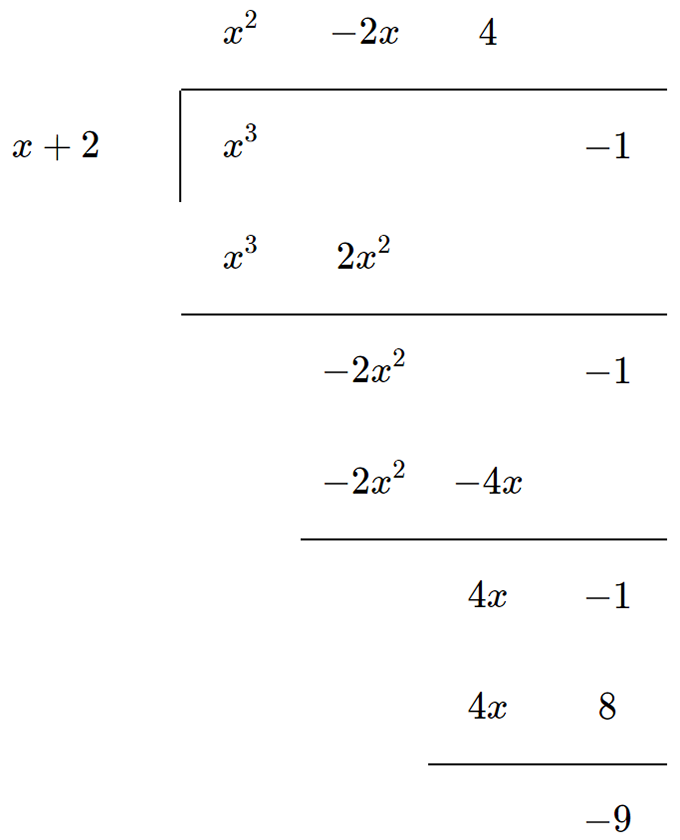#Álgebra: División de polinomios

1.
$$\frac{{x}^{3}-12{x}^{2}-42}{x-3}$$
Solución
2.
$$\frac{{x}^{3}-12{x}^{2}-42}{{x}^{2}+x-3}$$
Solución
3.
$$\frac{{x}^{3}-1}{x+2}$$
Solución
4.
$$\frac{3{x}^{3}-2{x}^{2}-3+4x}{3+3x+{x}^{2}}$$
Solución
5.
$$\frac{{x}^{3}+4{x}^{2}-9}{x+3}$$
Solución
6.
$$\frac{{x}^{3}+5x+2{x}^{2}+10}{x+2}$$
Solución
7.
$$\frac{{x}^{3}-4x+1}{{x}^{2}-4}$$
Solución
8.
$$\frac{{u}^{2}}{4+u}$$
Solución

# Polynomial Division - Introduction

When you see a polynomial that is a fraction composed of two polynomials — one as the numerator and the other as the denominator — it can often be simplified using long division. The procedure is based on the same principle of long division for whole numbers. Here is how it works.

# Recap on Polynomials

The word "polynomial" means "many terms" — something like
$$4x+2y-3$$
is a common polynomial form. Note that while polynomials can contain constants (such as
$$5$$
or
$$-17$$
), variables (such as
$$x$$
and
$$y$$
) and exponents, such as
$${x}^{2}$$
or
$${x}^{3}$$
, polynomials must not contain negative exponents or division by a variable such as
$$\frac{2}{x}$$
.

# An Example

Let’s try some polynomial division practice. Consider this polynomial:
$$\frac{{x}^{3}-1}{x+2}$$
First, we rewrite this as a form of long division. The only difference from regular long divisions is that, instead of numbers, they are polynomials.Step 1: Divide
$${x}^{3}$$
by
$$x$$
, which gives
$${x}^{2}$$
. Write this on the line above
$${x}^{3}$$
.
Step 2: Multiply
$${x}^{2}$$
by
$$x+2$$
, which gives
$${x}^{3}+2{x}^{2}$$
. Write this below
$${x}^{3}$$
.
Step 3: Subtract
$${x}^{3}+2{x}^{2}$$
from
$${x}^{3}$$
to give
$$-2{x}^{2}$$
. Write this below
$${x}^{3}+2{x}^{2}$$
and carry the
$$-1$$
as well.
Step 4: Divide
$$-2{x}^{2}$$
by
$$x$$
to give
$$-2x$$
. Write this next to
$${x}^{2}$$
above the line.
Step 5: Multiply
$$-2x$$
by
$$x+2$$
to give
$$-2{x}^{2}+-4x$$
. Write this below
$$-2{x}^{2}$$
.
Step 6: Subtract
$$-2{x}^{2}+-4x$$
from
$$-2{x}^{2}$$
to give
$$4x$$
. Write this below
$$-4x$$
and carry the
$$-1$$
again.
Step 7: Divide
$$4x$$
by
$$x$$
to give
$$4$$
. Write this next to
$$-2x$$
above the line.
Step 8: Multiply
$$4$$
by
$$x+2$$
to give
$$4x+8$$
. Write this below
$$4x+1$$
.
Step 9: Subtract
$$4x+8$$
from
$$4x-1$$
to give a remainder of
$$-9$$
.
Step 10: Write the final answer:
$$-{x}^{2}-2x+4-\frac{9}{x+2}$$

# What's Next

Interested in learning more about polynomial division? Start with our polynomial division problems at the top of this page. Our practice questions let you tackle problems at your own pace. If you get stumped, click on "Solution" to see exactly how we arrived at the answer. Want even more help? Sign up for Cymath Plus today.
At Cymath, it is our mission to help students tackle math concepts via practice. Try our online explanations and solutions to boost your math competence today!